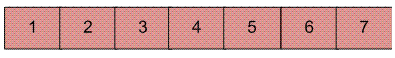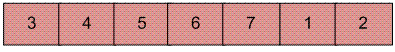# Java Program for Reversal algorithm for array rotation

• Difficulty Level : Easy
• Last Updated : 04 Dec, 2018

Write a function rotate(arr[], d, n) that rotates arr[] of size n by d elements.

Example :

```Input :  arr[] = [1, 2, 3, 4, 5, 6, 7]
d = 2
Output : arr[] = [3, 4, 5, 6, 7, 1, 2]
```Rotation of the above array by 2 will make array## Recommended: Please solve it on “PRACTICE ” first, before moving on to the solution.

Algorithm :

```rotate(arr[], d, n)
reverse(arr[], 1, d) ;
reverse(arr[], d + 1, n);
reverse(arr[], 1, n);
```

Let AB are the two parts of the input array where A = arr[0..d-1] and B = arr[d..n-1]. The idea of the algorithm is :

• Reverse A to get ArB, where Ar is reverse of A.
• Reverse B to get ArBr, where Br is reverse of B.
• Reverse all to get (ArBr) r = BA.

Example :
Let the array be arr[] = [1, 2, 3, 4, 5, 6, 7], d =2 and n = 7
A = [1, 2] and B = [3, 4, 5, 6, 7]

• Reverse A, we get ArB = [2, 1, 3, 4, 5, 6, 7]
• Reverse B, we get ArBr = [2, 1, 7, 6, 5, 4, 3]
• Reverse all, we get (ArBr)r = [3, 4, 5, 6, 7, 1, 2]

Below is the Java implementation of the above approach:

 `// Java program for reversal algorithm of array rotation``import` `java.io.*;`` ` `class` `LeftRotate {``    ``/* Function to left rotate arr[] of size n by d */``    ``static` `void` `leftRotate(``int` `arr[], ``int` `d)``    ``{``        ``int` `n = arr.length;``        ``rvereseArray(arr, ``0``, d - ``1``);``        ``rvereseArray(arr, d, n - ``1``);``        ``rvereseArray(arr, ``0``, n - ``1``);``    ``}`` ` `    ``/*Function to reverse arr[] from index start to end*/``    ``static` `void` `rvereseArray(``int` `arr[], ``int` `start, ``int` `end)``    ``{``        ``int` `temp;``        ``while` `(start < end) {``            ``temp = arr[start];``            ``arr[start] = arr[end];``            ``arr[end] = temp;``            ``start++;``            ``end--;``        ``}``    ``}`` ` `    ``/*UTILITY FUNCTIONS*/``    ``/* function to print an array */``    ``static` `void` `printArray(``int` `arr[])``    ``{``        ``for` `(``int` `i = ``0``; i < arr.length; i++)``            ``System.out.print(arr[i] + ``" "``);``    ``}`` ` `    ``/* Driver program to test above functions */``    ``public` `static` `void` `main(String[] args)``    ``{``        ``int` `arr[] = { ``1``, ``2``, ``3``, ``4``, ``5``, ``6``, ``7` `};``        ``leftRotate(arr, ``2``); ``// Rotate array by 2``        ``printArray(arr);``    ``}``}``/*This code is contributed by Devesh Agrawal*/`
Output:
```3 4 5 6 7 1 2
```

Time Complexity: O(n)

Please refer complete article on Reversal algorithm for array rotation for more details!

My Personal Notes arrow_drop_up